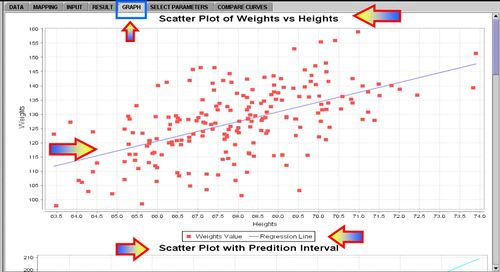# SOCR Events May2008 C9 S1

## SOCR May 2008 Event - Analyze bivariate data using linear regression methods

### Fit regression lines to pairs of numeric variables and calculate the means and standard deviations of the two variables and the correlation coefficient, using technology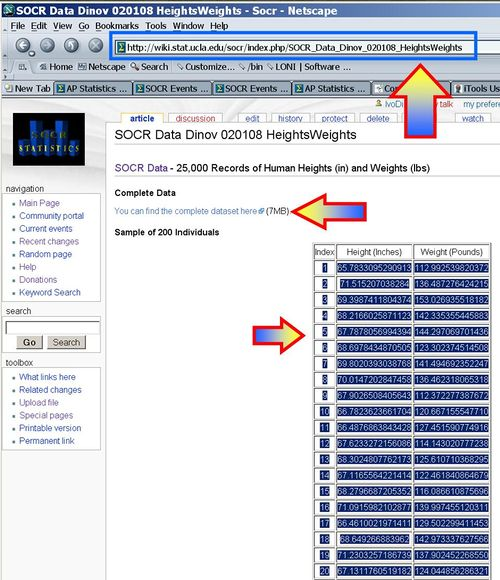• Paste these in SOCR and Map the Heights and Weights columns to the X and Y variables.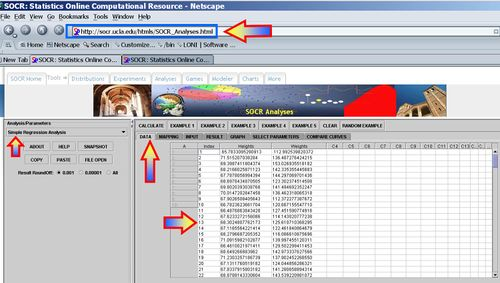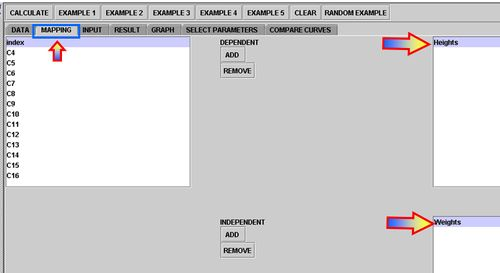• Click CALCULATE and see the output numerical results (in the Results tab) and the graphical outputs (in the Graphs tab).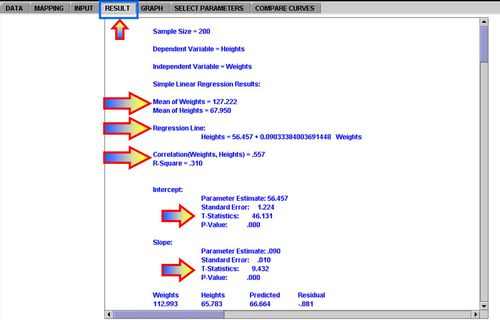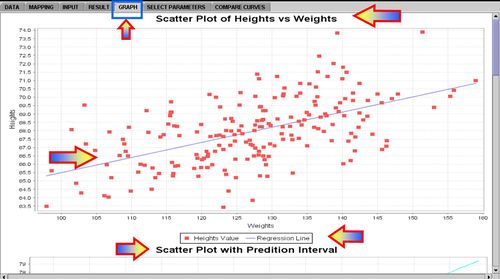### Compute predictions of y-values for given x-values using a regression equation, and recognize the limitations of such predictions

The regression line we computed and illustrated on the image above shows the following linear relation between Heights (inches) and Weights (pounds):

$$Height = 56.457 + 0.09033384003691448\times Weight$$

What would be the regression of the Weight on Height (the reverse regression)? You can compute this by hand to be

$$Weight = -105.959 + 3.431662594473845\times Height.$$

Or you can simply re-maps (swap) the dependent and independent variables in SOCR Analysis (Simple Linear Regression) and recalculate the linear relation.